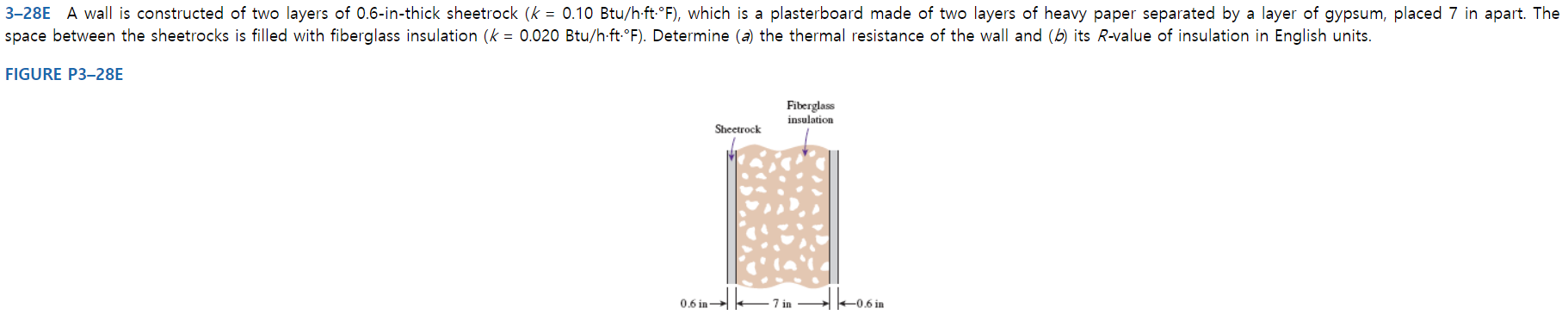# 3-28E A wall is constructed of two layers of 0.6-in-thick sheetrock (k = 0.10 Btu/h-ft-°F), which is a plasterboard made of two layers of heavy paper separated by a layer of gypsum, placed 7 in apart. Thespace between the sheetrocks is filled with fiberglass insulation (k = 0.020 Btu/h-ft F). Determine (a) the thermal resistance of the wall and (b) its R-value of insulation in English units.FIGURE P3-28EFiberglassinsulationSheetrock0.6 in7 inI 0.6 in

Question
30 viewshelp_outlineImage Transcriptionclose3-28E A wall is constructed of two layers of 0.6-in-thick sheetrock (k = 0.10 Btu/h-ft-°F), which is a plasterboard made of two layers of heavy paper separated by a layer of gypsum, placed 7 in apart. The space between the sheetrocks is filled with fiberglass insulation (k = 0.020 Btu/h-ft F). Determine (a) the thermal resistance of the wall and (b) its R-value of insulation in English units. FIGURE P3-28E Fiberglass insulation Sheetrock 0.6 in 7 in I 0.6 in fullscreen
check_circle

Step 1

Thickness of fiber glass insulation, L = 7 in.

Thermal conductivity of sheetrock Ks = 0.10 Btu/h. ft. °F

Thermal conductivity of insulation Ki = 0.020 Btu/h. ft. °F

Thickness of sheet rock L1 = 0.6 in.

Step 2

Part (a): The thermal resistance of the wall:

According to the diagram the thermal resistance of the wall is calculated as:

Here, L1 and L3 is the thickness of the sheet rock and L2 is the thickness of the fiber glass. And, k1 and k3 is the thermal conductivity of the sheet rock and k2 is the thermal conductivity of fiber glass.

Step 3

Thus, the total resistance of the wall is 30...

### Want to see the full answer?

See Solution

#### Want to see this answer and more?

Solutions are written by subject experts who are available 24/7. Questions are typically answered within 1 hour.*

See Solution
*Response times may vary by subject and question.
Tagged in

### Mechanical Engineering